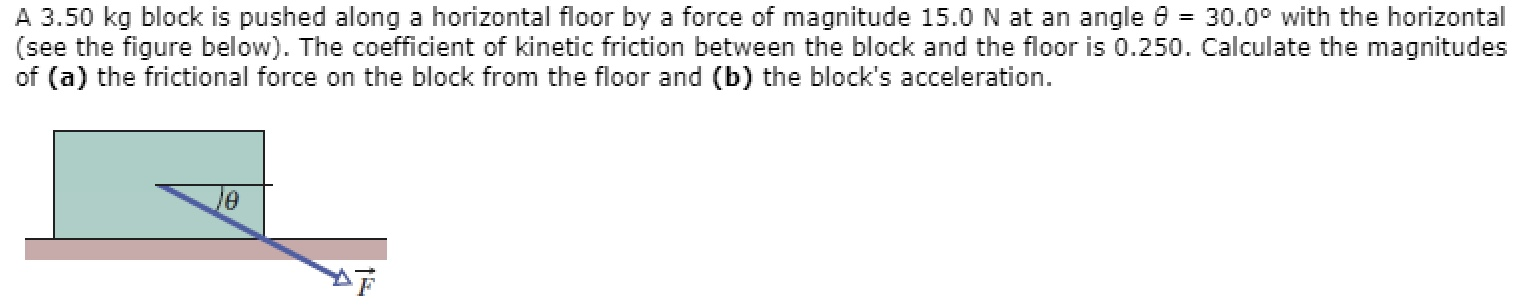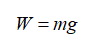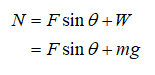# A 3.50 kg block is pushed along a horizontal floor by a force of magnitude 15.0 N at an angle 0 = 30.0° with the horizontal(see the figure below). The coefficient of kinetic friction between the block and the floor is 0.250. Calculate the magnitudesof (a) the frictional force on the block from the floor and (b) the block's acceleration.

Question
7 views

The four forces acting on the block must be identified.

Which forces act on the block?

Kinetic frictional force

Gravitational force

Pushing force

Static frictional force

Normal force

Tensionhelp_outlineImage TranscriptioncloseA 3.50 kg block is pushed along a horizontal floor by a force of magnitude 15.0 N at an angle 0 = 30.0° with the horizontal (see the figure below). The coefficient of kinetic friction between the block and the floor is 0.250. Calculate the magnitudes of (a) the frictional force on the block from the floor and (b) the block's acceleration. fullscreen
check_circle

The forces act on the block:

Normal force,

Kinetic friction force,

Pushing force,

Gravitational force

Part (a)

Weigh of the block,Step 3

Normal force,...

### Want to see the full answer?

See Solution

#### Want to see this answer and more?

Solutions are written by subject experts who are available 24/7. Questions are typically answered within 1 hour.*

See Solution
*Response times may vary by subject and question.
Tagged in

### Physics